Published: 15 November 2017

# The stability loss of the rotor-slide bearings system under random load variations

Paweł Pietkiewicz1
Sławomir Banaszek2
Grzegorz Żywica3
1University of Warmia and Mazury, Olsztyn, Poland
2, 3Institute of Fluid Flow Machinery, Polish Academy of Sciences, Gdańsk, Poland
Corresponding Author:
Grzegorz Żywica
Views 163

#### Abstract

The article presents how the system equipped with a rotor supported by slide bearings loses stability, operating under random load conditions. The computer simulations were applied for the analysis of the rotating machinery (using in-house developed codes). The MESWIR software is presented herein that can be used to assess dynamic performance of machines of this type. A special algorithm for the randomisation of loads was implemented and discussed in detail on the basis of a representative example. The rotor model was subjected to randomly-generated transverse forces during its operation. It exhibited unstable behaviour manifesting itself in the form of oil whirl or oil whip. The system operation was analysed both under constant and random load.

## 1. Introduction

Oil-lubricated bearings are commonly used in many types of rotating machinery, both large and small. They can support relatively high loads, but under certain conditions, they exhibit unstable operation, namely, the occurrence of oil whirl/whip. The presence of such phenomena is almost always accompanied by high-amplitude self-excited vibrations. The escalation of vibration amplitude may eventually lead to the machine damage, and can even be dangerous to the machine operator [1-3]. Although the stability loss of the rotor-bearing system is well described theoretically, the transition mechanisms between oil whirls and oil whips inside a bearing are not discovered yet. Unfortunately, the symptoms of an operational instability of oil-lubricated bearings are sometimes misunderstood, ignored, or falsely attributed to other causes. The continuing operational instability may amplify vibration and generate subharmonic spectral components, which should be recognised as the symptom of a defect by any diagnostic system. In order to model such phenomena, we need to use nonlinear methods [1-5].

It is commonly known that the high vibration levels, often accompanied by noise [6, 7], reflect some deviations from normal operation of a mechanical system. They may be caused by assembly errors or fluctuation of some operational parameters (which may be done on purpose in a numerical model or can happen in real life when operating rotating machinery) [8-10]. Such non-foreseeable problems can affect the measured signal, thus rendering the measurement results unreliable to some extent. Obviously, it is not difficult to imagine a situation where the aforementioned problems lead to drawing incorrect conclusions about the dynamical state of a machine [11, 12]. On the other hand, numerical computations are usually carried out using deterministic models, deterministic methods and deterministic data. The models of the loads existing in the machine and the machine itself are based on pre-established generalisations (perhaps idealised in some way), and therefore do not take into account any uncertainties. Of course, it is a common approach to simplify models and methods because it facilitates the analysis of the machine’s dynamic state. However, this also makes the calculation results do not fully reflect the physical processes taking place inside a machine. The inclusion of uncertainties in the calculation model directly affects the simulation results [13-15] and sometimes should be taken into account, preferably as early as possible in the design process [10, 16].

For a few years now, the scientists, during analysing and designing rotating machinery, have been devoting more and more attention to the problem of uncertainty of data. In large part, this is because modern machines are frequently pushed to their limits, usually as a result of the optimisation process . In such operating conditions, small changes in input parameters or properties of the construction materials can contribute to premature wear or maximum permissible stresses being exceeded and consequently create a major-accident hazard [11, 18]. Most of the research undertaken in this field has an analytical character. However, the research results that have been obtained so far have a considerable impact on the design process of rotating machinery and also on their final size and shape [19, 20]. It also seems that the research studies published in journals are constantly shaping and reshaping the scientific understanding of that subject.

The uncertainty of data pertains both to external forces acting on the rotating system and to properties of the system itself (its geometry, constructional features and material properties). The article  discusses the impact of stiffness and damping characteristics of a flexible support on the operational stability of a symmetrical rotor supported by gas bearings. It was shown that the bearing supports having the appropriate stiffness and damping coefficients can ensure stable operation of the rotor over a broad range of rotational speeds, yet even a small change in parameters of the support may lead to a severe deterioration of the operating conditions of this system. The impact of properties of a viscoelastic support on the dynamic state of a bent shaft was presented in the publication . The finite element method is used to model the system and the Lagrangian approach is applied to develop the equations of motion. On the basis of the obtained results, it was shown that mass and stiffness of the bearing supports have a major impact on the critical speed and overall vibration level. The main features of fluid-film bearings (i.e. oil viscosity and radial clearance) also significantly affect the dynamical performance of a rotating system. The uncertainty analysis of those parameters was presented in the article . The work described in  is focused on the dynamics of a nonlinear rotating system equipped with floating ring bearings and shows how far this system may be influenced by design parameters of the bearing itself. The performed analysis was based on the application of the bifurcation theory to the rotor model created on the basis of an exemplary rotor of the automotive turbocharger. The article  discusses the loss of dynamic stability of the rotor supported by tilting pad bearings, which occurred at speeds up to four times higher than the critical speed. The analysis conducted took into account the rotor unbalance and the bearings structure. The authors of the publication  investigated how a transverse crack of the rotating shaft affects its vibration level, taking into account the uncertainty of the physical parameters. The analysis also took into account changes in the shaft stiffness due to the crack. This was modelled using a random truncated Fourier series. The dynamic response of the system thus obtained was compared with the results of calculations carried out using the procedure being a combination of two methods: Harmonic Balance Method and Stochastic Finite Element Method (SFEM). Attempts are also being made to evaluate impacts of nonlinearities existing in rotating machinery on their dynamic properties. Examples of such analyses are given in the publications [5, 16, 26, 27]. The article  describes the analysis of a flexible rotor with a drill bit, taking into account stick-slip and time-delay aspects. In the paper , the effect of different linear and nonlinear random variables on the dynamic behaviour of the flexible rotor was examined in detail. Based on the results obtained in the research, the authors of the article  recommend introducing uncertainties in the design process in order to optimize the design.

Analyses of rotating machinery conducted in the last few years also took into account the variation of external forces acting on the system [14, 28] and various types of defects as well . The article  presents the research on the operational stability of the rotor which was subjected to stochastic axial loads. In the articles [31, 32], the impact of the changes in the magnitude of unbalance and its location on the dynamic characteristics of high-speed rotors was discussed. Without a doubt, damages such as blade loss , rubbing between rotating blade and casing, crack in the bearing support , disc offset  or shaft misalignment  have a major impact on the dynamic properties of rotating systems. During the analysis of a misaligned multi-supported rotor, the authors of the article  studied the uncertainty of several parameters such as misalignment as well as stiffness and damping of the bearing supports. It was concluded that the increase in data uncertainty causes the rise in vibration amplitudes of the rotor.

On the basis of the literature review, one can definitely say that the uncertainty of some parameters during the analysis of rotating machinery should be taken into account since the values of these parameters can have a strong impact on the obtained results. To the best of our knowledge, this paper is the first to study the dynamics of a rotor supported by slide bearings, which was subjected to random transverse forces. Such load conditions can occur during operation of various machines, e.g. in belt transmissions (where the belt tension is continuously changing), with a partial immersion of the rotor elements in a lubricating liquid or during changes in the generator’s load. This article discusses the research on the effect of uncertainty in the external load on the dynamic performance of the rotating system. It shows the results of the computational analysis in which the sub-harmonic vibration development and the unstable machine operation were observed. The random radial forces variations were considered in order to apply some uncertainty in the form of a variable centrifugal force as an exemplary operational parameter. Then, the comparison of the results obtained from both models (fully deterministic and randomized) is presented.

## 2. Description of the numerical model

For the purposes of the analysis, the in-house developed computer programs (which make up the MESWIR software) were used. This part of the article discusses the main features and capabilities of the software. The method of modelling the bearings and the rotor was presented, and the calculation algorithm as well. In order to carry out the research discussed herein, some of the computer programs had to be modified accordingly, as explained in the following part of the article.

The software called MESWIR was created at the Institute of Fluid-Flow Machinery, Polish Academy of Sciences. This software is the fruit of many years’ work, begun some time ago by a team of scientists under the leadership of Professor Jan Kiciński [2-4]. It allows to conduct various types of analyses of rotating systems and has effective graphical tools for pre- and post-processing. It makes possible to model different operational states of a rotor-bearings system, taking into account the mutual interactions between the machine elements. The nonlinear computational model implemented in this software is very useful since it provides the user with the vibration history that can be further transformed into the vibration trajectories and vibration spectra. On the basis of such results, we can obtain information about whether or not the test machine operation is stable.

### 2.1. Numerical model of the bearing

The proposed model is, in fact, composed of two separate models: the beam model of the shaft and the model of the hydrodynamic slide bearings. Fig. 1 presents the general model of the rotor with bearings, supports, discs, external dampers, etc. The shaft model is digitized using beam finite elements. The unbalance and the other external loads are applied to the nodes. Fig. 2 shows the way of modelling a hydrodynamic slide bearing. Generally, oil-lubricated bearings have anisotropic properties, because the pairs of stiffness and damping main coefficient are not equal, i.e. ${c}_{11}\ne {c}_{22}$ and ${d}_{11}\ne {d}_{22}$. Moreover, in contrast to a classical linear mechanical system, the cross-coupled coefficients are not equal (${c}_{12}\ne {c}_{21}$ and ${d}_{12}\ne {d}_{21}$) and they usually take values different from zero.

On the other hand, stiffness and damping coefficients depend on the journal position. In dynamical analyses, these coefficients are also affected by the rate of change of the journal position. The nonlinear nature of the system consisting of a rotor supported by oil-lubricated bearings results from the cross-coupled coefficients features that were mentioned above and from their time-dependence as well.

Fig. 1The exemplary mechanical system of the rotating machine with applied forces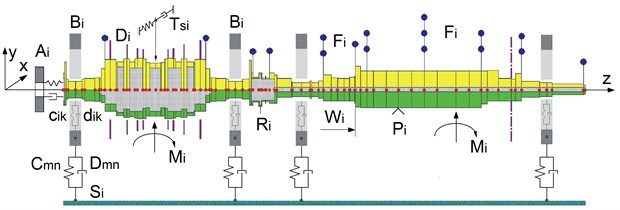Fig. 2The mechanical and thermal models of a journal bearing ; cik and dik – stiffness and damping coefficients, OC – centre of the journal, OP – centre of the bearing bush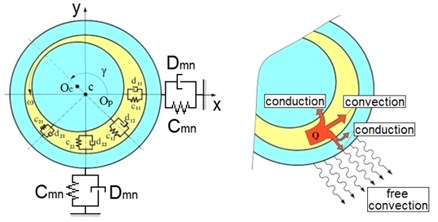In the first calculation step, the kinetostatic analysis is conducted. During this analysis, the static loads are applied to the shaft and the rotor rotates at a fixed speed. This is needed to determine basic characteristics of the slide bearings.

From the mathematical point of view, the influence of bearings on the rotor can be described by means of four stiffness coefficients and four damping coefficients of the oil film:

1
${c}_{11}=\frac{\partial {W}_{x}}{\partial x},{c}_{22}=\frac{\partial {W}_{y}}{\partial y},{c}_{12}=\frac{\partial {W}_{x}}{\partial y},{c}_{21}=\frac{\partial {W}_{y}}{\partial x},$
2
${d}_{11}=\frac{\partial {W}_{x}}{\partial \stackrel{˙}{x}},{d}_{22}=\frac{\partial {W}_{y}}{\partial \stackrel{˙}{y}},{d}_{12}=\frac{\partial {W}_{x}}{\partial \stackrel{˙}{y}},{d}_{21}=\frac{\partial {W}_{y}}{\partial \stackrel{˙}{x}},$
3
$\left\{\begin{array}{l}{c}_{ik}\\ {d}_{ik}\end{array}\right\=f\left(\epsilon ,\gamma ,\stackrel{˙}{\epsilon },\stackrel{˙}{\gamma }\right),$

where ${W}_{x}$, ${W}_{y}$ are the components of the oil film reaction, $\epsilon$ is the eccentricity of the shaft centre within the bearing clearance (non-dimensional quantity, related to the clearance gap) and $\gamma$ denotes the angular position of the journal. The dots over the symbols indicate the time derivatives. They can be obtained as the solution of the following three-dimensional Reynolds equation:

4
$\frac{\partial }{\partial x}\left(\frac{\partial p}{\partial x}A\right)+\frac{\partial }{\partial z}\left(\frac{\partial p}{\partial z}A\right)=-\left[{U}_{1}\left(\frac{\partial h}{\partial x}-\frac{\partial B}{\partial x}\right)+\frac{\partial h}{\partial \tau }\right],$

where:

$A=-\frac{{h}^{3}}{12\mu },B=\frac{1}{2}h.$

where $p$ – oil pressure, $h$ – oil film thickness, $\mu$ – lubricant viscosity, ${U}_{1}$ – linear velocity of a bearing journal, $\tau$ – time, $A$, $B$ – functions of the viscosity and the local film thickness.

The Reynolds equation, i.e. Eq. (4), describes the oil pressure distribution. It is solved with the following boundary conditions: the oil pressure at the edge of the bearing is equal to the ambient pressure, the pressure in the supply pocket is equal to the supply pressure and the end of the oil film is defined by the Swift-Stieber condition. The Swift-Stieber condition describes the end of the oil film where both pressure and pressure gradient become zero or equal to cavitation pressure. In practice, this is the most frequently used boundary condition for the Reynolds equation (also known as Reynolds boundary condition), and it describes the location of the film-cavity interface . The system of equations obtained in this way is solved iteratively using the Successive Overrelaxation (SOR) method .

Fig. 3The calculation flowchart for a slide bearing (t – temperature, μ – viscosity)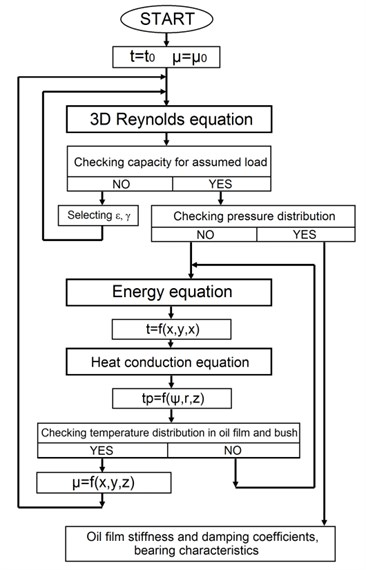The thermal conditions of the bearing are described by means of the energy equation and the heat conduction equation. The energy equation defines the temperature distribution in the lubricating film. It takes into account the oil heating caused by the shear between the lubricant layers inside the bearing gap. The heat conduction equation describes the temperature distribution in the bearing bush and the bearing journal. The particular forms of equations and their solutions are presented in publication .

### 2.2. Numerical model of the rotor

The dynamic analysis of the rotor is carried out using the computer program called NLDW which is a part of the MESWIR software. Basically, the calculation method is based on the FEM and the rotor line is modelled with beam finite elements. It generates time histories for the selected nodes of the analysed rotor model.

The calculation algorithm is based on the well-known equation of motion:

5
$M\stackrel{¨}{q}+D\left(q,\stackrel{˙}{q}\right)\stackrel{˙}{q}+K\left(q,\stackrel{˙}{q}\right)q=p\left(\tau \right),$

where $M$ – mass matrix, $D$ – damping matrix, $K$ – stiffness matrix, $P$ – vector of excitation forces, $q$, $\stackrel{˙}{q}$, $\stackrel{¨}{q}$ – vectors of generalized displacements, velocities and accelerations, $\tau$ – time.

Eq. (5) is a matrix equation describing the motion of the entire rotating system and it is composed of a number of mutually coupled differential equations. Their amount is equal to the number of degrees of freedom in the system. All matrices are created in local coordinate systems (individually for each finite element) and then they are transformed into the global coordinate system.

Fig. 4A beam finite element with 6 DOFs and its local coordinate system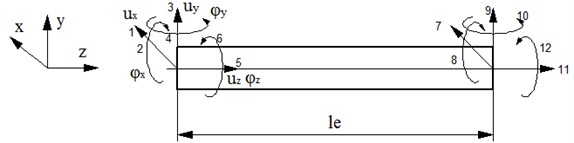In order to model the rotor, a beam finite element with 6 DOFs per node is created in a local coordinate system (see Fig. 4). For each element one has to build the following matrices:

• stiffness matrix of the beam element ${K}_{e}$,

• inertia matrix of the beam element ${M}_{e}$,

• damping matrix of the beam element ${D}_{e}$,

• damping matrix generated by the Coriolis forces ${D}_{ce}$,

• inertia matrix of the disk ${M}_{d}$,

• gyroscopic matrix of the disk ${D}_{gd}$,

• stiffness and damping matrices of the slide bearings ${K}_{le}$, ${D}_{le}$,

• stiffness, damping and inertia matrices of the foundation ${K}_{ef}$, ${D}_{ef}$, ${M}_{ef}$.

The above matrices are transformed into the global coordinate system.

The stiffness matrix of the element is built using the flexibility matrix and the appropriate transformation matrices:

6
${K}_{e}={T}^{t}{L}^{-1}T,$

where ${K}_{e}$ – stiffness matrix of the element, $T$ – matrix of transformation from the dependent to the independent system of forces, $L$ – flexibility matrix of the element, $t$ – denotation for a transposed matrix, −1 – denotation for an inverse matrix.

The transformation matrix is derived from the static equilibrium conditions with the assumption that the nodal forces in the first node are linearly independent and the forces in the second node are described as functions of the first ones. The whole matrix has the following form:

7
$T=\left[\begin{array}{cccccccccccc}-1& {l}_{e}& 0& 0& 0& 0& 1& 0& 0& 0& 0& 0\\ 0& -1& 0& 0& 0& 0& 0& 1& 0& 0& 0& 0\\ 0& 0& -1& -{l}_{e}& 0& 0& 0& 0& 1& 0& 0& 0\\ 0& 0& 0& -1& 0& 0& 0& 0& 0& 1& 0& 0\\ 0& 0& 0& 0& -1& 0& 0& 0& 0& 0& 1& 0\\ 0& 0& 0& 0& 0& -1& 0& 0& 0& 0& 0& 1\end{array}\right],$

where ${l}_{e}$ – element length.

One may calculate the flexibility matrix using the elastic strain energy, according to the following formula:

8
$U=\underset{0}{\overset{{l}_{e}}{\int }}\frac{{N}^{2}dz}{2ES}+\underset{0}{\overset{{l}_{e}}{\int }}\frac{{M}_{x}^{2}dz}{2E{I}_{x}}+\underset{0}{\overset{{l}_{e}}{\int }}\frac{{M}_{y}^{2}dz}{2E{I}_{y}}+\underset{0}{\overset{{l}_{e}}{\int }}\frac{{M}_{s}^{2}dz}{2G{I}_{s}}+\underset{0}{\overset{{l}_{e}}{\int }}\frac{\beta {}_{x}{}^{}{P}_{x}^{2}dz}{2GS}+\underset{0}{\overset{{l}_{e}}{\int }}\frac{{\beta }_{y}{P}_{y}^{2}dz}{2GS},$

where ${l}_{e}$ – element length, $S$ – cross-section area, ${I}_{x}$ – second moment of area according to the $x$ axis, ${I}_{y}$ – second moment of area according to the $y$ axis, ${I}_{s}$ – polar moment of area, ${\beta }_{x}$ – cross-section shear coefficient in the $x$ direction, ${\beta }_{y}$ – cross-section shear coefficient in the $y$ direction, $E$ – Young’s modulus, $G$ – Kirchhoff’s modulus, $N$, $P$, $M$ – forces and moments in a beam cross-section (axial force, shearing force and bending moment).

The forces and moments can be expressed as functions of independent nodal forces according to the following equilibrium equations:

9
${P}_{x}={P}_{1},{P}_{y}={P}_{3},N={P}_{5},{M}_{x}={P}_{1}z+{P}_{4},{M}_{y}={P}_{3}z-{P}_{2}$${M}_{s}={P}_{6},$

where ${P}_{1}$, ${P}_{2}$, ..., ${P}_{6}$ are the forces related to particular degrees of freedom in the node (see Fig. 4).

By inserting the above expressions in the elastic strain energy formula and taking into account the independent nodal forces we obtain the symmetric flexibility matrix :

10
$L=\left[\begin{array}{cccccc}\frac{{l}_{e}^{3}}{3EI}+\frac{\beta {l}_{e}}{GS}& \frac{{l}_{e}^{2}}{2EI}& 0& 0& 0& 0\\ \frac{{l}_{e}^{2}}{2EI}& \frac{{l}_{e}}{EI}& 0& 0& 0& 0\\ 0& 0& \frac{{l}_{e}^{3}}{3EI}+\frac{\beta {l}_{e}}{GS}& \frac{{l}_{e}^{2}}{2EI}& 0& 0\\ 0& 0& \frac{{l}_{e}^{2}}{2EI}& \frac{{l}_{e}}{EI}& 0& 0\\ 0& 0& 0& 0& \frac{{l}_{e}}{ES}& 0\\ 0& 0& 0& 0& 0& \frac{{l}_{e}}{G{I}_{S}}\end{array}\right],$

where:

11
$I={I}_{x}={I}_{y}.$

The fact that matrices $D$ and $K$ depend on the parameters of motion ($q$, $\stackrel{˙}{q}$) implies the structural nonlinearity of the system. The mass, damping and stiffness matrices (respectively $M$, $D$ and $K$) include all mutually coupled characteristic submatrices related to the main subsystems of the structure. It is practically impossible to extract them in an unambiguous manner due to the nonlinear form of Eq. (5). In the iteration procedure, at each time step the global matrices $D$ and $K$ are updated by new values of stiffness and damping coefficients ${c}_{ik}$ and ${d}_{ik}$ of oil-lubricated bearings and also by new additional stiffness parameters resulting from defects of the rotor (if any) .

The general calculation scheme of the computer program called NLDW is presented in Fig. 5. The symbol $\phi$ denotes the shape of the deflection of the rotor line (a set of the deflection angles at individual nodes), which is the sum of kinetostatic and dynamic deflection lines (${\phi }_{k}$ and ${\phi }_{d}$, respectively).

## 3. The object of research

The analysed Jeffcott rotor is supported by two cylindrical slide bearings (Fig. 6). The steel rotor has the length of 1.4 m and its diameter is 0.1 m. The whole rotor weighs 280 kg, while the disc weighs 185 kg. The nominal diameter of the bearings is 0.1 m and is the same as the outer diameter of the rotor. The length of each bearing is 0.05 m, L/D ratio is 0.5 and the radial clearance is 90 μm. The oil having the viscosity of 0.02 Ns/m was used as a lubricant. It flows through two inlets into the oil clearance.

Fig. 5Schematic illustration of the calculation process implemented in the computer program called NLDW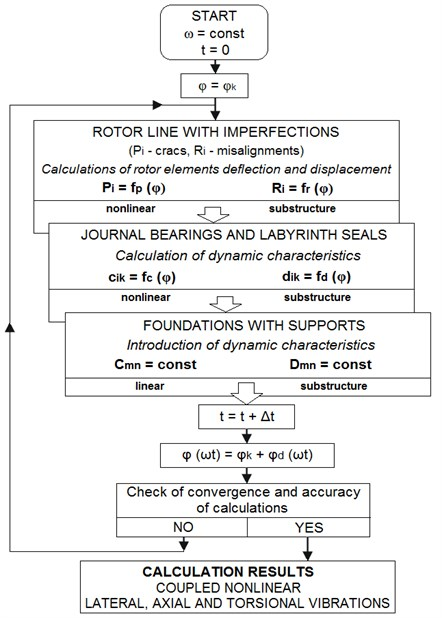The selection of the object of research was based on experience gained to date. The test rotor is symmetrical and has a rather simple geometry. It greatly facilitates interpreting calculation results and formulating conclusions about the dynamical behaviour of this machine. Despite the simple structure of the rotor and the bearings, a sophisticated numerical model of the mechanical system was used, which has already been described in the previous part of this article. For the purposes of this research, the model was extended to contain various types of external forces, including the possibility to change force values during each time step of the simulation of rotor operation. This issue is discussed further in the next part of the article.

## 4. Randomisation of the force

The lateral force $P$ was applied to the centre of the rotor. It was equidistant to both bearings, as shown in Fig. 6. The base value of the force is equivalent to that of a centrifugal force of the residual unbalance mass of 0.0257 kg on the radius of 0.18 m. The rotor rotated at a speed up to 7.000 rpm. Fig. 7 shows the nominal values of force $P$ as a function of rotational speed of the rotor.

The force direction undergoes periodic changes synchronously with the rotational movement of the rotor. The base magnitude of the force depends on the rotational speed, as it was shown in Fig. 7. Additionally, it varies randomly. The variance may be up to 20 percent, plus or minus.

Fig. 6Model of the analysed rotor with its discretisation and the lateral force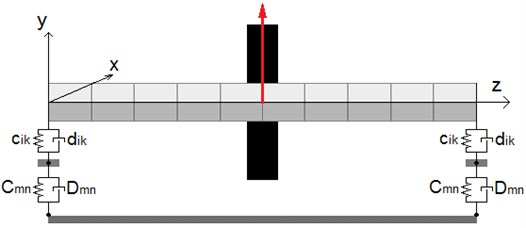Fig. 7Lateral force P vs. rotational speed of the rotor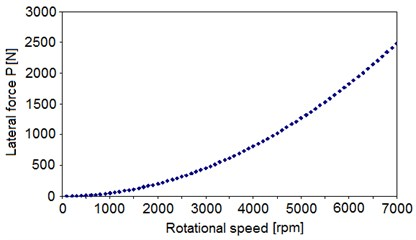Fig. 8The exemplary values of force P obtained during calculations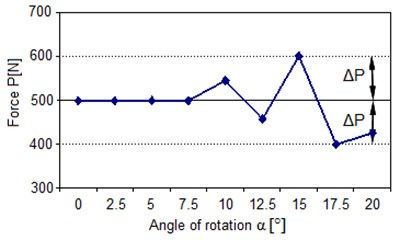The instantaneous force is calculated at each time step using $k-1$ algorithm, created at the University of Warmia and Mazury [37-39]. The randomisation of the force $P$ involves changing its magnitude by ±20 % of the base value. After the randomisation, the lateral force may change its value from 80 percent to 120 percent in comparison to the deterministic value that is fixed for each rotational speed.

The $k-1$ algorithm is carried out through the following four steps:

1) Generation of a sign (+1 or –1).

2) Generation of a number denoted by $\mathrm{\Delta }P$1 within the range of <0, 20>.

3) Calculation of the value of $\mathrm{\Delta }P$, according to the following formula: $\mathrm{\Delta }P=\mathrm{s}\mathrm{i}\mathrm{g}\mathrm{n}·\mathrm{\Delta }P1%·P$.

4) Calculation of a random value of the centrifugal force, according to the following formula: ${P}_{rand}=P+\mathrm{\Delta }P$.

The exemplary values of force $P$, obtained at subsequent calculation steps, are shown in Fig. 8. It shows the case in which force $P$ varies randomly within the range of [500-100, 500+100].

## 5. Calculation results

Fig. 9 presents the amplitudes of lateral vibrations of the disc centre as a function of rotational speed of the rotor. The rotor vibrations have been forced by the load mentioned above. The research was conducted for the rotor rotational speeds within the range from 0 to 5.700 rpm. The value of 5.700 rpm is higher than the rotor’s critical speed. The calculation results for the base case, i.e. for the case in which the ratio $x$ of the force ${P}_{rand}$ to force $P$ at each rotational speed of the rotor is equal to 1 $\left(x=$ 1; $\mathrm{\Delta }P=$ 0). The randomized value of the force is generated with the use of the k-1 algorithm described in the previous section. Both results are compared and presented in Fig. 9. The two curves presented on the graph show the vibration amplitudes for the system that was excited using deterministic and randomized forces ($P$ and ${P}_{rand}$, respectively). The random forces are calculated by the algorithm described above. In the base case, forces $P$ and ${P}_{rand}$ equal each other. Some minor differences in the results occur only within narrow speed ranges. The reason for that lies in the nature of the system – nonlinear behaviour of the bearings.

Fig. 10 presents exemplary trajectories of the bearing journal and their corresponding vibration spectra for selected rotational speeds. The selected calculation results show the significant changes in trajectory shapes, leading to the stability loss of the rotating system. The calculations were carried out under the assumption that the rotational speeds have to be changed in small steps in order to be able to effectively capture the moment in which a stability loss occurs (in other words: to effectively capture the transient states between different trajectory shapes).

Fig. 9Vibration amplitudes vs. rotational speed of the rotor (the base case)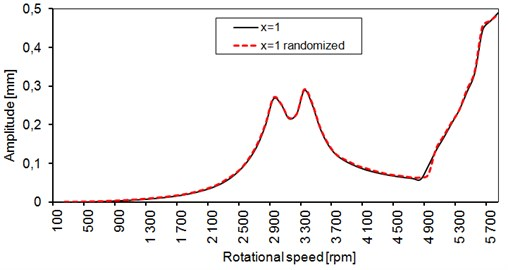Some of the issues relating to the stability loss of rotating machinery are described in the publications [1-4]. One can distinguish a few phases of the process leading to the stability loss. In the initial phase, the vibration trajectories take the shape of a loop. Then, two ellipses can be observed. Subsequently, one of them gets smaller as the rotational speed increases. The speed of that precession is the half of a revolution. The FFT analysis shows that the subharmonic components ($h/$2) appear in the vibration spectra ($h$ means a component synchronous with the rotor speed). They are clearly visible in Fig. 10(a) and 10(b). The vibration amplitudes increase rapidly, and after two revolutions, the journal moves in the entire bearing clearance. This phase is called “oil whirl”.

The second phase is a transient phase. The oil whirls disappear but the amplitudes continue to rise. The trajectory shape may resemble or give the general impression of a stable operation of the bearing. The amplitude of the $h/$2 component continues to increase and becomes dominant as the rotational speed increases (Fig. 10(d)). The second ellipse (loop) disappeared (Fig. 10(e)), but the vibration amplitude is still relatively large, and the $h/$2 component still dominates.

At about 5.400 rpm, the trajectory once again takes elliptical shape (Fig. 10(f)) but the rotational speed of the precession does not change. The vibration amplitude begins to rise as the $h/$2 component increases. This is the consequence of the oil whirls, which can generate self-excited vibrations due to the presence of high hydrodynamic forces in the lubricating film [2, 3]. The presented vibration spectra show the frequency of those vibrations at 50 Hz. It can lead to self-excited resonance vibrations. In such situations, only the bearing clearance limits their amplitude. The vibration spectrum in Fig. 10(g) is the situation like this. The vibration amplitude is much higher than in the previous cases.

The following figures show the influence of the force randomisation on the development of instability. The vibration trajectories of the bearing journal are shown and compared. Each trajectory is presented on a square-shaped graph and a square’s width and height are equal, so the aspect ratio is 1:1. In the lower parts of the graphs, they are shown enlarged (with aspect ratio maintained) in order to expose all the details of the trajectories. The left trajectories were generated using the force randomisation, while the right ones were calculated without it. The amplitude characteristic is shown, and the red vertical line is a marker indicating the rotational speed at which both calculations were carried out.

Fig. 10Vibration trajectories and vibration spectra showing the stability loss in the bearing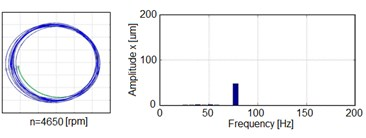a) Stable operation at 3.650 rpm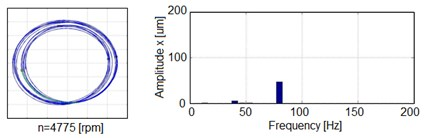b) Stability threshold at 4.775 rpm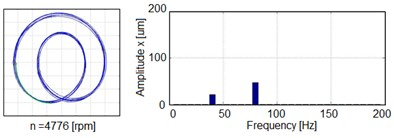c) Development of the instability at 4.776 rpm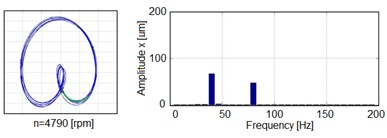d) Oil whirls at 4.790 rpm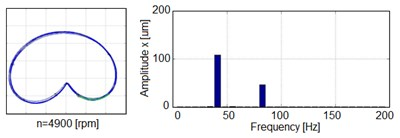e) Development of the oil whirls at 4.900 rpm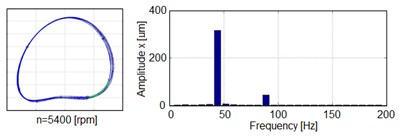f) Development of the oil whirls at 5.400 rpm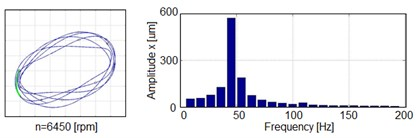g) Oil whip at 6.450 rpm

Figs. 11 and 12 show the comparison of vibration trajectories in the first phase of the stability loss. The range of rotational speed of this phase is relatively narrow. The trajectory in Fig. 12 looks like two rings placed close to each other. The additional $h/$2 loop, relating to vibrations at the half of the harmonic frequency (see Fig. 10(c) and 10(d)) appears and disappears due to the increase in rotational speed. It seems that the system tries to return to the stable state. The moment in which the second form of the oil whirls appears is shown in Fig. 12. It is noteworthy that it appears for the first time in the case of random transverse force (Fig. 11).

Fig. 11The build-up of the oil whirls at 4.775 rpm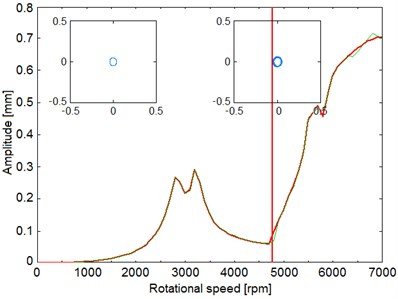Fig. 12The build-up of the oil whirls at 4.776 rpm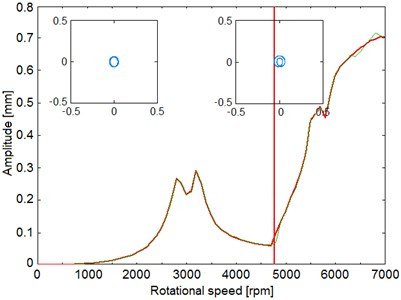At the rotational speed of ca. 4.790 rpm and for the constant transverse force, one can notice that the vibration trajectory maintains its shape, whether or not the transverse force is randomly-generated (see Fig. 12 and Fig. 13).

The trajectory which is shown in Fig. 14 forms itself gradually into a circle as rotational speed increases. Then, due to the gradual increase in rotational speed from 4.790 rpm to 4.950 rpm, one can observe that the system tries to return to the form of vibration with the internal loop. These changes are less intensive in the case with a random load.

Fig. 13The appearance of the second form of the oil whirls at 4.790 rpm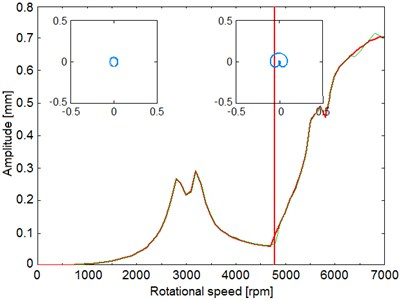Fig. 14The third phase of the oil whirls at 4.835 rpm the internal loop disappears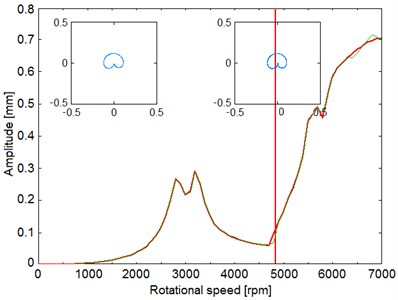Fig. 15Gradually fading concavity in the lower part of the vibration trajectory at 4.900 rpm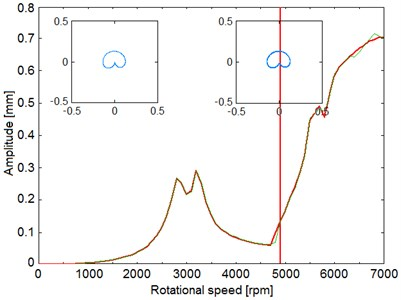Fig. 16Gradually fading concavity in the lower part of the vibration trajectory at 5.450 rpm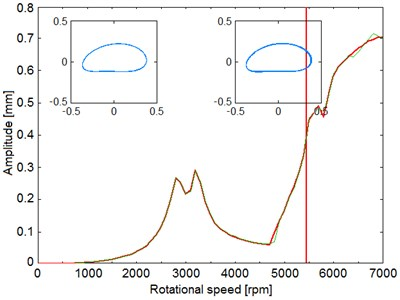Fig. 17Illustration showing the oil whip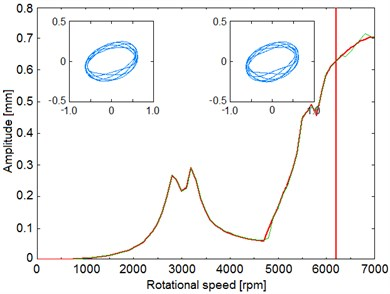After the system exceeds 4.900 rpm, the characteristic concavity in the lower part of the vibration trajectory begins to fade (Figs. 15 and Fig. 16). This process is more stable in the case with a random load. In the case of deterministic load, the rotor operation is not stable, and it returns once again to the previous form of vibration.

After exceeding 6.000 rpm, the system enters the last phase of the stability loss. The trajectory shape becomes chaotic and the vibration amplitude continues to rise rapidly (Fig. 17). In this phase, one cannot observe any differences between both cases of the rotor loading.

## 6. Conclusions

The presented research aimed at examining the possibilities of modelling the phenomena that are extremely important to people who design and maintain turbomachinery. A model capable of analysing the dynamical performance of a rotating machine was created, which made it possible to take a closer look at the phenomena that may appear in a mechanical system consisting of the rotor supported by slide bearings [2, 3]. In our model, the analysis of stability loss for a system in which the loads are randomly-generated is a novelty.

The comparison of the results of numerical simulations, obtained for the transverse force of a deterministic or random value, leads to the conclusion that certain phenomena relating to the stability loss can occur in different moments of machine operation depending on whether the values of load forces are randomly-generated or not.

The obtained results show that the assumption of some uncertainty in the value of the operational parameter (like the centrifugal force herein) can affect the operation of the rotor supported by slide bearings. Such uncertainties coupled with the nonlinear nature of slide bearings can increase vibrations and their subharmonic components. These differences can be significant, especially if the instability is in its initial phase. In the further phases of the instability, the differences decrease slightly.

The presented calculation results may form the basis for further research in this field, where another random force can be taken into consideration. The differences between the two load cases (deterministic and random) can be fundamentally important for the purposes of creating the inverse models. Such models may be necessary for clarifying ambiguity or similarity of obtained results.

The test rig, which allows running experiments aimed at verifying the results obtained through numerical calculations, was built at the Department of Mechanics and Fundamentals of Machinery Design, University of Warmia and Mazury in Olsztyn. The results of such verification will be presented and discussed in the following publications.

#### Cited by

A state-of-the-art review on uncertainty analysis of rotor systems